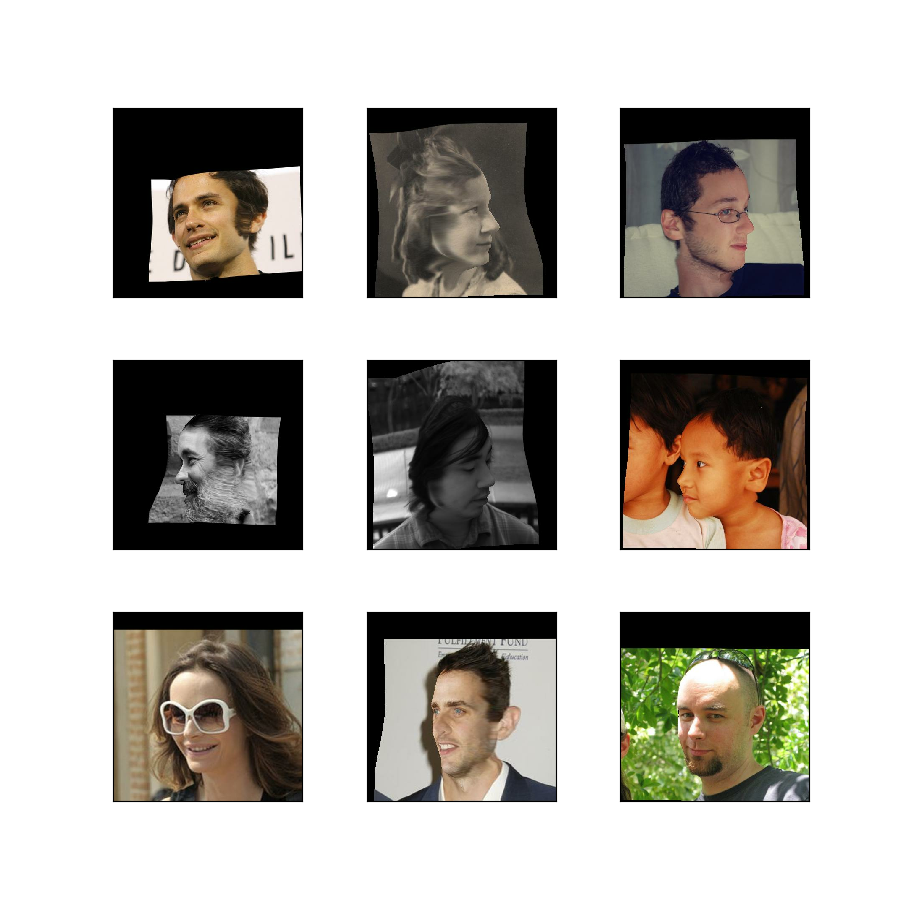# the300w_lp

• Description:

300W-LP Dataset is expanded from 300W, which standardises multiple alignment databases with 68 landmarks, including AFW, LFPW, HELEN, IBUG and XM2VTS. With 300W, 300W-LP adopt the proposed face profiling to generate 61,225 samples across large poses (1,786 from IBUG, 5,207 from AFW, 16,556 from LFPW and 37,676 from HELEN, XM2VTS is not used).

The dataset can be employed as the training set for the following computer vision tasks: face attribute recognition and landmark (or facial part) locaization.

Split Examples
`'train'` 61,225
• Features:
``````FeaturesDict({
'color_params': Tensor(shape=(7,), dtype=tf.float32),
'exp_params': Tensor(shape=(29,), dtype=tf.float32),
'illum_params': Tensor(shape=(10,), dtype=tf.float32),
'image': Image(shape=(450, 450, 3), dtype=tf.uint8),
'landmarks_2d': Tensor(shape=(68, 2), dtype=tf.float32),
'landmarks_3d': Tensor(shape=(68, 2), dtype=tf.float32),
'landmarks_origin': Tensor(shape=(68, 2), dtype=tf.float32),
'pose_params': Tensor(shape=(7,), dtype=tf.float32),
'roi': Tensor(shape=(4,), dtype=tf.float32),
'shape_params': Tensor(shape=(199,), dtype=tf.float32),
'tex_params': Tensor(shape=(199,), dtype=tf.float32),
})
``````
``````@article{DBLP:journals/corr/ZhuLLSL15,
author    = {Xiangyu Zhu and
Zhen Lei and
Xiaoming Liu and
Hailin Shi and
Stan Z. Li},
title     = {Face Alignment Across Large Poses: {A} 3D Solution},
journal   = {CoRR},
volume    = {abs/1511.07212},
year      = {2015},
url       = {http://arxiv.org/abs/1511.07212},
archivePrefix = {arXiv},
eprint    = {1511.07212},
timestamp = {Mon, 13 Aug 2018 16:48:23 +0200},
biburl    = {https://dblp.org/rec/bib/journals/corr/ZhuLLSL15},
bibsource = {dblp computer science bibliography, https://dblp.org}
}
``````